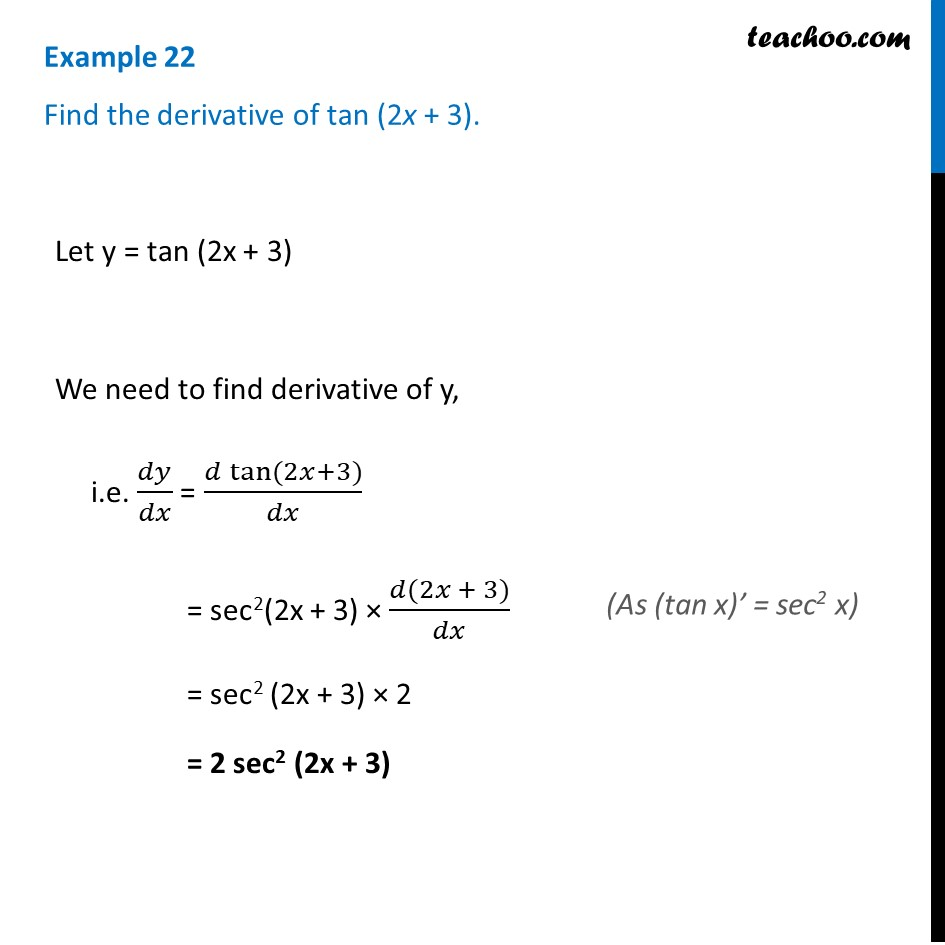Finding derivative of a function by chain rule

Chapter 5 Class 12 Continuity and Differentiability (Term 1)
Concept wise### Transcript

Example 22 Find the derivative of tan (2x + 3). Let y = tan (2x + 3) We need to find derivative of y, i.e. 𝑑𝑦/𝑑𝑥 = (𝑑 tan⁡〖(2𝑥+3)〗)/𝑑𝑥 = sec2(2x + 3) × (𝑑(2𝑥 + 3))/𝑑𝑥 = sec2 (2x + 3) × 2 = 2 sec2 (2x + 3) (As (tan x)’ = sec2 x)